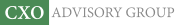Objective research to aid investing decisions

#### Value Investing Strategy (Strategy Overview)

Allocations for December 2023 (Final)
Cash TLT LQD SPY

#### Momentum Investing Strategy (Strategy Overview)

Allocations for December 2023 (Final)
1st ETF 2nd ETF 3rd ETF

# Mean-Variance Optimization Versus Equal Weight

| | Posted in: Strategic Allocation

Is equal weighting of diversified portfolio assets good enough, or are mean-variance optimized allocation strategies constructed from asset return and variance forecasts worth the complexities of implementation? In the June 2012 draft of their paper entitled “Market Volatility, Optimal Portfolios and Naive Asset Allocations”, Massimiliano Caporin and Loriana Pelizzon investigate the conditions under which mean-variance optimized portfolios outperform an equal-weight portfolio. They consider four optimized allocation strategies (mean-variance, and three variations of global minimum variance with no shorting). They apply these strategies to five sets of equity assets diversified across different U.S. stock anomalies according to: (1) industry; (2) size/value; (3) size/short-term reversal; (4) size/momentum; and, (5) size/long-term reversal. They consider four methods for forecasting returns and volatilities for these assets (lagged rolling values, regression on explanatory variables and two technical autoregressions that detect mean reversion), all based on a 60-month or 120-month rolling historical window. They use Sharpe ratio to compare portfolio performances. Using monthly data for the five selected sets of equity assets from Kenneth French’s library spanning April 1953 through December 2010 (693 months), they find that:

• Over the entire sample period, some mean-variance optimization strategies outperform equal weighting, but outperformance depends on the asset set, the optimization model applied and the return/volatility forecast window size. Optimization strategies work best when the return/volatility forecast method includes detection of mean reversion in asset returns.
• Whether or not mean-variance optimization strategies outperform equal weighting varies by subperiod, according to the observed degree of mean reversion within the subperiod.
• Outperformance of mean-variance optimization strategies concentrates in asset sets that do not impound mean reversion (such as size/value and size/momentum). Equal weighting often outperforms in asset sets that do impound mean reversion (such as size/short-term reversal).
• Return and volatility prediction errors tend to be large during high-volatility subperiods, such that mean-variance optimization strategies do not outperform equal weighting during times of market turbulence.

In summary, evidence regarding the relative risk-adjusted performance of various mean-variance optimization and equal weight asset allocation strategies is mixed.

Cautions regarding findings include:

• The study apparently uses gross returns. Differences among strategies in trading frictions for periodic portfolio rebalancing (not addressed in the paper) may lead to different findings on a net basis. Also, the costs of data collection and processing would disfavor the mean-variance optimization portfolios.
• Consideration of so many optimization strategy variations introduces data snooping bias, such that the best-performing strategies likely overstate future performance.
• This version of the paper is a rough draft, with no tables or figures.